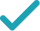Type a math problemSolve for xSteps Using the Quadratic Formula
Steps for Completing the Square
All equations of the form can be solved using the quadratic formula: . The quadratic formula gives two solutions, one when is addition and one when it is subtraction.
This equation is in standard form: . Substitute for , for , and for in the quadratic formula, .
Square .
Multiply times .
Multiply times .
Take the square root of .
Multiply times .
Now solve the equation when is plus. Add to .
Divide by .
Now solve the equation when is minus. Subtract from .
Divide by .
The equation is now solved.
Solve for ySteps for Solving Linear Equation
Subtract from both sides. Anything subtracted from zero gives its negation.
Subtract from both sides.
Subtract from both sides.
Divide both sides by .
Dividing by undoes the multiplication by .
Divide by .
Solve for x (complex solution)GraphGiving is as easy as 1, 2, 3
Get 1,000 points to donate to a school of your choice when you join Give With Bing
2x^{2}+8x+8-y=0
All equations of the form ax^{2}+bx+c=0 can be solved using the quadratic formula: \frac{-b±\sqrt{b^{2}-4ac}}{2a}. The quadratic formula gives two solutions, one when ± is addition and one when it is subtraction.
x=\frac{-8±\sqrt{8^{2}-4\times 2\left(8-y\right)}}{2\times 2}
This equation is in standard form: ax^{2}+bx+c=0. Substitute 2 for a, 8 for b, and -y+8 for c in the quadratic formula, \frac{-b±\sqrt{b^{2}-4ac}}{2a}.
x=\frac{-8±\sqrt{64-4\times 2\left(8-y\right)}}{2\times 2}
Square 8.
x=\frac{-8±\sqrt{64-8\left(8-y\right)}}{2\times 2}
Multiply -4 times 2.
x=\frac{-8±\sqrt{64+8y-64}}{2\times 2}
Multiply -8 times -y+8.
x=\frac{-8±\sqrt{8y}}{2\times 2}
x=\frac{-8±2\sqrt{2y}}{2\times 2}
Take the square root of 8y.
x=\frac{-8±2\sqrt{2y}}{4}
Multiply 2 times 2.
x=\frac{2\sqrt{2y}-8}{4}
Now solve the equation x=\frac{-8±2\sqrt{2y}}{4} when ± is plus. Add -8 to 2\sqrt{2y}.
x=\frac{\sqrt{2y}}{2}-2
Divide -8+2\sqrt{2y} by 4.
x=\frac{-2\sqrt{2y}-8}{4}
Now solve the equation x=\frac{-8±2\sqrt{2y}}{4} when ± is minus. Subtract 2\sqrt{2y} from -8.
x=-\frac{\sqrt{2y}}{2}-2
Divide -8-2\sqrt{2y} by 4.
x=\frac{\sqrt{2y}}{2}-2 x=-\frac{\sqrt{2y}}{2}-2
The equation is now solved.
2x^{2}+8x+8-y=0
Quadratic equations such as this one can be solved by completing the square. In order to complete the square, the equation must first be in the form x^{2}+bx=c.
2x^{2}+8x+8-y-\left(8-y\right)=-\left(8-y\right)
Subtract -y+8 from both sides of the equation.
2x^{2}+8x=-\left(8-y\right)
Subtracting -y+8 from itself leaves 0.
2x^{2}+8x=y-8
Subtract -y+8 from 0.
\frac{2x^{2}+8x}{2}=\frac{y-8}{2}
Divide both sides by 2.
x^{2}+\frac{8}{2}x=\frac{y-8}{2}
Dividing by 2 undoes the multiplication by 2.
x^{2}+4x=\frac{y-8}{2}
Divide 8 by 2.
x^{2}+4x=\frac{y}{2}-4
Divide y-8 by 2.
x^{2}+4x+2^{2}=\frac{y}{2}-4+2^{2}
Divide 4, the coefficient of the x term, by 2 to get 2. Then add the square of 2 to both sides of the equation. This step makes the left hand side of the equation a perfect square.
x^{2}+4x+4=\frac{y}{2}-4+4
Square 2.
x^{2}+4x+4=\frac{y}{2}
\left(x+2\right)^{2}=\frac{y}{2}
Factor x^{2}+4x+4. In general, when x^{2}+bx+c is a perfect square, it can always be factored as \left(x+\frac{b}{2}\right)^{2}.
\sqrt{\left(x+2\right)^{2}}=\sqrt{\frac{y}{2}}
Take the square root of both sides of the equation.
x+2=\frac{\sqrt{2y}}{2} x+2=-\frac{\sqrt{2y}}{2}
Simplify.
x=\frac{\sqrt{2y}}{2}-2 x=-\frac{\sqrt{2y}}{2}-2
Subtract 2 from both sides of the equation.
8x-y+8=-2x^{2}
Subtract 2x^{2} from both sides. Anything subtracted from zero gives its negation.
-y+8=-2x^{2}-8x
Subtract 8x from both sides.
-y=-2x^{2}-8x-8
Subtract 8 from both sides.
\frac{-y}{-1}=-\frac{2\left(x+2\right)^{2}}{-1}
Divide both sides by -1.
y=-\frac{2\left(x+2\right)^{2}}{-1}
Dividing by -1 undoes the multiplication by -1.
y=2\left(x+2\right)^{2}
Divide -2\left(2+x\right)^{2} by -1.